# Texas Go Math Grade 5 Lesson 1.3 Answer Key Place Value of Decimals

Refer to our Texas Go Math Grade 5 Answer Key Pdf to score good marks in the exams. Test yourself by practicing the problems from Texas Go Math Grade 5 Lesson 1.3 Answer Key Place Value of Decimals.

## Texas Go Math Grade 5 Lesson 1.3 Answer Key Place Value of Decimals

Unlock the Problem

The Brooklyn Battery Tunnel in New York City is 1.726 miles long. It is the longest underwater tunnel for vehicles in the United States. To understand this distance, you need to understand the place value of each digit in 1.726.You can use a place-value chart to understand decimals. Whole numbers are to the left of the decimal point. Decimals are to the right of the decimal point. The thousandths place is to the right of the hundredths place.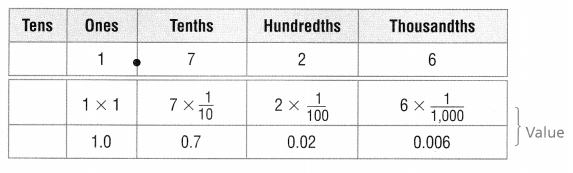The place value of the digit 6 in 1.726 is thousandths. The value of 6 in 1.726 is 6 $$\frac{1}{1,000}$$ or 0.006.
Standard Form: 1.726
Word Form: one and seven hundred twenty-six thousandths
Expanded Form: 1 + 0.7 + 0.02 + 0.006

Math Talk
Mathematical Processes

Explain how the value of the last digit in a decimal can help you read a decimal.
We know that,
Values to the left of the decimal point are greater than one.
Now,
38 means 3 tens and 8 ones.
The word name of the decimal is determined by the place value of the digit in the last place.
Ex:
In 1.3215,
The last digit (5) is in the ten-thousandth place

Try This!

Use place value to read and write decimals.

A. Standard Form: 2.35
Word Form: two and ____________
Expanded Form: 2 + ___________
The given standard form is: 2.35
Now,
We know that,
Expanded form means writing the number so you show the value of each digit. Word form means writing the number with words instead of numerals
So,
The word from of 2.35 is: Two and Thirty-five hundredths
The Expanded form of 2.35 is: 2 + 0.3 + 0.05
Hence, from the above,
We can conclude that
The word from of 2.35 is: Two and Thirty-five hundredths
The Expanded form of 2.35 is: 2 + 0.3 + 0.05

B. Standard Form: ____________
Word Form: three and six hundred fourteen thousandths
Expanded Form: ____________ + 0.6 + ____________ + ____________
The given word form is: Three and six hundred fourteen thousandths
Now,
We know that,
The standard form is the combination of digits written together as a single number. In the expanded form the digits of the number are split into each of the individual digits with their place value and written in expanded form
So,
The standard form of the given word form is: 3.614
The expanded form of the given word form is: 3 + 0.6 + 0.01 + 0.004
Hence, from the above,
We can conclude that
The standard form of the given word form is: 3.614
The expanded form of the given word form is: 3 + 0.6 + 0.01 + 0.004

Example

Use place value to compare.
The silk spun by a common garden spider is about 0.003 mm thick. A commonly used sewing thread is about 0.3 mm thick. How does the thickness of the spider silk and the thread compare?
Count the number of decimal place-value positions to the digit 3 in 0.3 and 0.003.
0.3 has 2 fewer decimal places than 0.003
2 fewer decimal places: 10 × 10 = 100
So,
0.3 is 100 times as much as 0.003
0.003 is 0.01 of 0.3
So, the thread is 0.01 times as thick as the garden spider’s silk.
So,
The thickness of the garden spider’s silk is 0.01 times that of the thread.

Share and Show

Complete the place-value chart to find the value of each digit.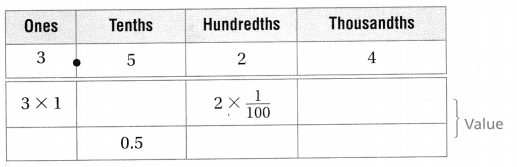The given number is: 3.524
Hence,
The completed place-value chart for the given number is:Write the value of the underlined digit.

Question 2.
0.543
The given number is: 0.543
So,
The representation of the given number in the place-value table is: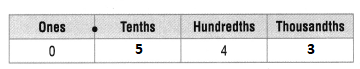Hence, from the above,
We can conclude that
The value of the underlined digit in 0.543 is: 4 Hundredths

Question 3.
6.234
The given number is: 6.234
So,
The representation of the given number in the place-value table is: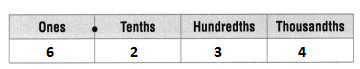Hence, from the above,
We can conclude that
The value of the underlined digit in 6.234 is: 2 Tenths

Question 4.
3.954
The given number is: 3.954
So,
The representation of the given number in the place-value table is:Hence, from the above,
We can conclude that
The value of the underlined digit in 3.954 is: 4 Thousandths

Write the number in two other forms.

Go Math 5th Grade Lesson 1.3 Express as Decimal Numerals Question 5.
0.253
The given number is: 0.253
Now,
From the given number,
We can observe that
The given number is in standard form
So,
The Word form of 0.253 is: Two hundred and fifty-three thousandths
The Expanded form of 0.253 is: 0 + 0.2 + 0.05 + 0.003
Hence, from the above,
We can conclude that
The representation of the given number in other two forms is:
The Word form of 0.253 is: Two hundred and fifty-three thousandths
The Expanded form of 0.253 is: 0 + 0.2 + 0.05 + 0.003

Question 6.
7.632
The given number is: 7.632
Now,
From the given number,
We can observe that
The given number is in standard form
So,
The Word form of 7.632 is: Seven and six hundred and thirty-two thousandths
The Expanded form of 7.632 is: 7 + 0.6 + 0.03 + 0.002
Hence, from the above,
We can conclude that
The representation of the given number in other two forms is:
The Word form of 7.632 is: Seven and six hundred and thirty-two thousandths
The Expanded form of 7.632 is: 7 + 0.6 + 0.03 + 0.002

Problem Solving

Question 7.
Connect You can use place-value patterns to rename a decimal. Complete the chart to rename 0.3 using other place values.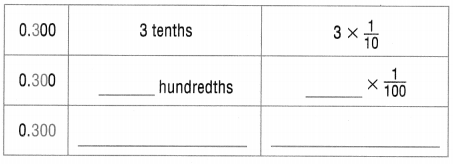The given number is: 0.3
So,
From the given number,
We can observe that
There is 30 hundredths and 300 thousandths place value
Hence,
The complete place-value chart for 0.3 using other place values is: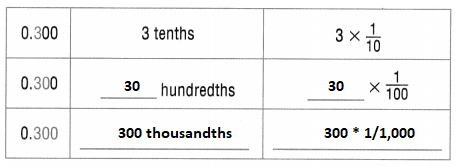Write Math
Explain how you know that the digit 6 in the numbers 3.675 and 3.756 does not have the same value.
The given decimal numbers are: 3.675 and 3.756
So,
The representation of 3.675 in the place-value table is: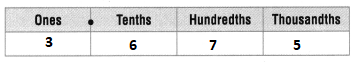So,
The representation of 3.756 in the place-value table is: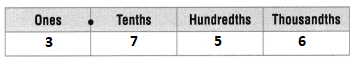Hence, from the above,
We can conclude that
The value of the digit 6 from 3.675 and 3.756 does not have the same value by using the place-value tables

Problem Solving

Use the table for 9-11.Question 9.
What is the value of the digit 7 in New Mexico’s average annual rainfall?
The given table is:Now,
From the given table,
We can observe that
The average rainfall in New Mexico is: 0.372 meters
Now,
The representation of New Mexico’s rainfall in the place-value table is: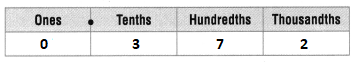Hence, from the above,
We can conclude that
The value of the digit 7 in New Mexico’s average rainfall is: 7 hundredths

Lesson 1.3 5th Grade Go Math Understand Decimal Place Value Question 10.
The average annual rainfall in Maine is one and seventy-four thousandths meters per year. Complete the table by writing that amount in standard form.
It is given that
The average annual rainfall in Maine is one and seventy-four thousandths meters per year
Now,
The representation of the given word form in the standard form is: 1.074 meters
Hence, from the above,
We can conclude that
The complete table with Maine’s average rainfall is:Question 11.
Which of the states has an average annual rainfall with the least number in the thousandth place?
From Exercise 10,
We know that,
The complete table with Maine’s average rainfall is:Now,
When we compare the thousandths place in all the numbers,
We can observe that
0.820 has the least value in thousandths place
Hence, from the above,
We can conclude that
Wisconsin has the average rainfall with the least number in the thousandths place

Question 12.
H.O.T. Multi-Step Dan used a meter stick to measure some seedlings in his garden. One day, a corn stalk was 0.85 m tall. A tomato plant was 0.850 m. A carrot top was 0.085 m. Which plant was shortest?
It is given that
Dan used a meter stick to measure some seedlings in his garden. One day, a corn stalk was 0.85 m tall. A tomato plant was 0.850 m. A carrot top was 0.085 m
So,
The representation of 0.85 in the place value table is:So,
The representation of 0.850 in the place value table is: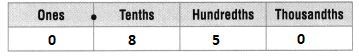So,
The representation of 0.085 in the place value table is: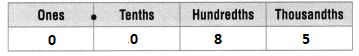Now,
When we compare the tenths position of the three numbers,
We can observe that
0.8 = 0.850 > 0.085
Hence, from the above,
We can conclude that
A carrot top has the shortest length

Go Math 5th Grade Lesson 1.3 Understand Decimal Place Value Question 13.
H.O.T. What’s the Error? Damian wrote the number four and twenty-three thousandths as 4.23. Describe and correct his error.
It is given that
Damian wrote the number four and twenty-three thousandths as 4.23
Now,
The representation of the given word form in the standard form is: 4.023
Now,
The representation of Damian’s number in the place value chart is: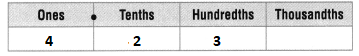Now,
The actual representation of Damian’s number in the place value chart is:Now,
When we compare the place value charts,
We can observe that
Damian’s number does not have thousandths place but he mentioned thousandths in his number’s word form
Hence, from the above,
We can conclude that
Damian’s error is: The misrepresentation of the word form into the correct standard form
The correct representation of Damian’s number is: 4.023

Question 14.
Although the average turkey sandwich in Turkeyton costs 3.456 dollhorts, the cost of a gourmet turkey sandwich at Sandwich Palace is 5.032 dollhorts. What does the 3 in 5.032 dollhorts represent?
A. 3 dollhorts
B. 3 tenths of a dollhort
C. 3 hundredths of a dollhort
D. 4 hundredths of a dollhort
It is given that
Although the average turkey sandwich in Turkeyton costs 3.456 dollhorts, the cost of a gourmet turkey sandwich at Sandwich Palace is 5.032 dollhorts.
Now,
The representation of 5.032 in the place value chart is:Hence, from the above,
We can conclude that
The digit 3 in 5.032 represents:Question 15.
Use Math Language One meter is equal to about 3.281 feet. What is the word form of this decimal?
A. three and twenty-eight hundredths and one thousandth
B. three and two hundred eighty-one thousandths
C. three and two tenths and eighty-one thousandths
D. three point two eighty-one
It is given that
One meter is equal to about 3.281 feet
Hence, from the above,
We can conclude that
The representation of 3.281 feet in the word form is:Go Math Grade 5 Lesson 1.3 Understand Decimal Place Value Question 16.
Multi-Step Sam had a piece of ribbon 8.469 cm long. He cut off exactly one-tenth of a cm from the ribbon. Then he cut off two-hundredths of a cm. How long is the ribbon now?
A. 8.169 cm
B. 8.269 cm
C. 8.349 cm
D. 8.466 cm
It is given that
Sam had a piece of ribbon 8.469 cm long. He cut off exactly one-tenth of a cm from the ribbon. Then he cut off two-hundredths of a cm
Now,
The present length of the ribbon = 8.469 – 0.1 – 0.02
= 8.349 cm
Hence, from the above,
We can conclude that
The length of the ribbon now is:Texas Test Prep

Question 17.
In 24.736, which digit is in the thousandths place?
A. 4
B. 7
C. 3
D. 6
The given number is: 24.736
Now,
The representation of 24.736 in the place value chart is: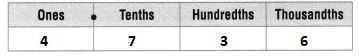Hence, from the above,
We can conclude that
The digit that is present in thousandths place in 24.736 is:### Texas Go Math Grade 5 Lesson 1.3 Homework and Practice Answer Key

Rename 0.6 using other place values.

Question 1.The given number is: 0.6
Now,
When we observe the given number,
We can say that there is 60 hundredths and 600 thousandths in the given number
Hence,
The complete table of 0.6 using other place values is: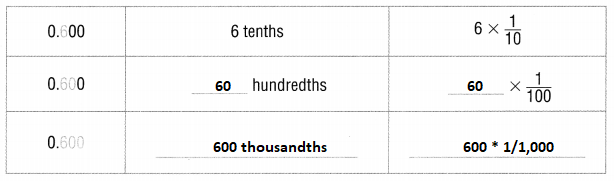Rename 0.2 using other place values.

Question 2.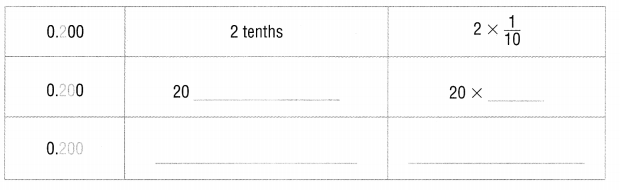The given number is: 0.2
Now,
When we observe the given number,
We can say that there is 20 hundredths and 200 thousandths in the given number
Hence,
The complete table of 0.6 using other place values is:Write the number in two other forms.

Question 3.
0.632
The given number is: 0.632
Now,
From the given number,
We can observe that
The given number is in standard form
So,
The Word form of 0.632 is: Six hundred and thirty-two thousandths
The Expanded form of 0.253 is: 0 + 0.6 + 0.03 + 0.002
Hence, from the above,
We can conclude that
The representation of the given number in other two forms is:
The Word form of 0.632 is: Six hundred and thirty-two thousandths
The Expanded form of 0.253 is: 0 + 0.6 + 0.03 + 0.002

Question 4.
4.293
The given number is: 4.293
Now,
From the given number,
We can observe that
The given number is in standard form
So,
The Word form of 4.293 is: Four and two hundred and ninety-three thousandths
The Expanded form of 4.293 is: 4 + 0.2 + 0.09 + 0.003
Hence, from the above,
We can conclude that
The representation of the given number in other two forms is:
The Word form of 4.293 is: Four and two hundred and ninety-three thousandths
The Expanded form of 4.293 is: 4 + 0.2 + 0.09 + 0.003

Problem Solving

Question 5.
A weather reporter reads aloud the number of meters of snowfall. The amount is 0.103 meter. What words should the reporter use on the air to explain the amount of snowfall?
It is given that
A weather reporter reads aloud the number of meters of snowfall. The amount is 0.103 meter
So,
The representation of 0.103 in the Word form is: One hundred and three thousandths
Hence, from the above,
We can conclude that
The words the reporter should use on the air to explain the amount of snowfall is: One hundred and three-thousandths meters

Homework and Practice 1.3 Decimals to Thousandths Answer Key Question 6.
Amil says that the snow on the ground is 5 × $$\frac{1}{100}$$ meter high. How can this number be expressed as a decimal?
It is given that
Amil says that the snow on the ground is 5 × $$\frac{1}{100}$$ meter high
Now,
5 × $$\frac{1}{100}$$ = 5 × 0.01
= 0.05 meters
Hence, from the above,
We can conclude that
The representation of 5 × $$\frac{1}{100}$$ as a decimal is: 0.05

Lesson Check

Question 7.
In the number 5.923, which number is in the thousandths place?
A. 5
B. 9
C. 2
D. 3
The given number is: 5.923
Now,
The representation of 5.923 in the place-value chart is:Hence, from the above,
We can conclude that
The digit that is present in the thousandths place in the given number is: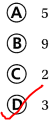Question 8.
Which number has the same value as 7 × $$\frac{1}{1,000}$$ ?
A. 0.7
B. 0.007
C. 0.07
D. 7.0
The given expression is: 7 × $$\frac{1}{1,000}$$
Now,
7 × $$\frac{1}{1,000}$$ = 7 × 0.001
= 0.007
Hence, from the above,
We can conclude that
The number that has the same value as 7 × $$\frac{1}{1,000}$$ is:Question 9.
Multi-Step Which value correctly represents the number that is 10 times as great as five hundred eighty-one thousandths?
A. 5.81
B. 581
C. 0.581
D. 500.81
The given Word form is: five hundred eighty-one thousandths
So,
The representation of the given Word form in the standard form is: 0.581
Now,
According to the given information,
10 × 0.581 = 5.81
Hence, from the above,
We can conclude that
The correct value that represents the number that is 10 times as great as five hundred eighty-one thousandths is:Question 10.
Which number has the same value as 3 × $$\frac{1}{10}$$?
A. 0.003
B. 3.10
C. 0.3
D. 0.03
The given expression is: 3 × $$\frac{1}{10}$$
So,
3 × $$\frac{1}{10}$$ = 3 × 0.1
= 0.3
Hence, from the above,
We can conclude that
The number that has the same value as 3 × $$\frac{1}{10}$$ is:Question 11.
Jack’s baby sister crawled three and five tenths meters today. Which number represents that distance?
A. 3.5
B. 3.05
C. 3.510
D. 3.005
It is given that
Jack’s baby sister crawled three and five tenths meters today
So,
The representation of the given Word form in the Standard form is: 3.5 meters
Hence, from the above,
We can conclude that
The number that represents the Jack’s baby sister’s crawling distance is:Question 12.
Multi-Step Leon measured the distance each of his four toy cars rolled across the floor. Which car measurement that has a 4 in the thousandths place represents the shortest distance rolled?
A. 0.741 meter
B. 0.714 meter
C. 0.224 meter
D. 0.213 meter
It is given that
Leon measured the distance each of his four toy cars rolled across the floor.
So,
From the given options,
We can observe that
There are two options that have 4 in the thousandths place.
They are: 0.714 meters and 0.224 meters
Now,
From the above numbers,
We can observe that
The shortest number is: 0.224 meters
Hence, from the above,
We can conclude that
The car measurement that has a 4 in the thousandths place and represents the shortest distance rolled is: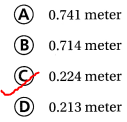Scroll to Top